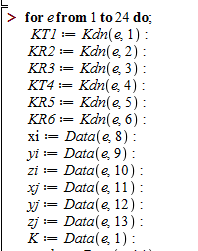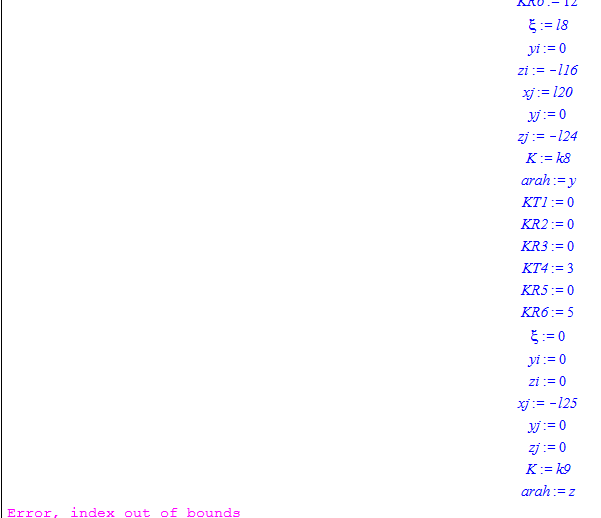## How can I increase the 1000000 limit of output...

Please is it possible to increase the 1000000 limit of output? I tried doing some computation in form of power series for S10 but I got the message "1000000 limit of output exceeced". How can I resolve this?

## out of bound cases...

i got some trouble when i tried to build large matrix. in my case, notification error out of bound appear when looping stop at 9 from 24 repeatation.and this is my looping command:the result of the script was:## different asymptotics using asympt and Multiseries...

Hey guys,

I have the following occurence:

ii_inf:=x^(2-s)*(x^(-s)*GAMMA(3-s)*GAMMA(2-2*s)/(GAMMA(2-s)*GAMMA(3-2*s))+x^(s-2)*GAMMA(3-s)*GAMMA(2*s-2)/GAMMA(s))/(2-s)+(1/2)*(2*s*x-x+1)*(x+1)/(((x+1)^s)^2*(2*s^2-3*s+1))+x^(1-s)*(x^(-s)*GAMMA(2-s)*GAMMA(-2*s+1)/(GAMMA(1-s)*GAMMA(2-2*s))+x^(-1+s)*GAMMA(2-s)*GAMMA(2*s-1)/GAMMA(s))/(1-s)+(x+1)/((2*s-1)*((x+1)^s)^2)+x/((-1+s)*x^s)-(x+1)/((-1+s)*(x+1)^s);

ii_inf=simplify(ii_inf);

asympt(ii_inf,x,3);

Multiseries:-asympt(ii_inf,x,1);
gives different results...the last one however seems to be the correct one...

What is happening here?

## Plotting an expression with unknown function Gener...

Hello, I am trying to plot an expression for my General Relativity class, this expression is one of the expanding universe FLRW solutions to the Einstein field equations and contains an unknown functions.My question is how do I get maple to plot this?

Thank you

## Calculating integral...

If I calculate the integral:

z:=exp(I*t)

evalf(Int(z^(1/2)*(diff(z, t)), t = 0 .. 2*Pi))

I get -1.33333333*I

If I calculate

int(z^(1/2)*(diff(z, t)), t = 0 .. 2*Pi)

I get -4/3

so where is the I coming from? Am I doing sth  something   wrong?

I might add: if I calculate the same for z:=0.5+exp(I*t) I get 0 and -I*0.4714....

so what is going different here?

## How can I do iteration in Maple?...

 >>>>(1)
 >>(2)(3)Error, unterminated for loopError, missing numerator>>>>>>>>>>(1)
 >>(2)(3)Error, unterminated for loopError, missing numerator>>>